Previous: 2.1.2 Poisson Equation Up: 2.1 The Boltzmann Poisson Next: 2.1.4 Distribution Function Models

## 2.1.3 Dimensional Reduction

To derive a numerically tractable model from the full (3+3)-dimensional Boltzmann equation we assume that the distribution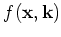is one-dimensional in space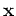and has cylinder symmetry in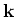. That iswhere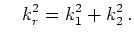(2.11)

This is known as the 1+2''-Boltzmann equation. Note that also the input data has to fulfill this symmetry. Consequently the cylinder radial components of the electrical field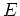vanish.

With this assumption we have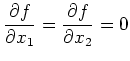(2.12)

and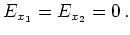(2.13)

Hence the BTE reduces for parabolic bands to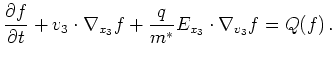(2.14)